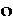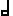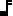A Binary Bottle for Binary Wine

We will present here a new Rhythmic Code (Rcode for short)
which applies to each note of a piece of music,
and which defines, clearly and accurately,
the exact position of the note,
the precise length of the note
(its Duration, the Quantitative Dimension of TIME), and
the quality of Beat/Offbeat of the note at all levels
(its Alternation, the Qualitative Dimension of TIME).

Fractions in the Binary Code
To assure precise and abbreviated identification of these three parameters
(position, duration, and alternation)
we will use the binary system of numerical notation (with only "0"s and "1"s).
This is common practice for units -
1=1, 10=2, 11=3, 100=4, and so on,
each column representing an exponential of 2,
as they do for exponentials of 10 in the decimal system.
We will "extend" the system to include fractions,
with numerals ("0" or "1") which will follow the "binary point"
as we commonly do with the decimal point -
.1=1/2, .01=1/4, .11=3/4, and so on,
each column now representing a negative exponential of 2,
as they do for the negative exponentials of 10 in the decimal system,
and, above all, a specific rhythmic level.

Coding Note-values
The choice of note-values is evidently arbitrary, and this Rcode could be adjusted to any choice.
However, we will remain here in the choice which is the most convenient
- the complete bar of Level -1 will be composed of a whole-note ("")
and will be placed just before the binary point:
1. will represent the complete first bar,
10. will represent the complete second bar,
11. will represent the complete third bar,
100. will represent the complete fourth bar, and so on...
For bars of larger size, the "*" will be used to include both 0 and 1 at that level.
1*. will represent the first bar of Level 0
which includes both the second (10.) and third (11.) bars of Level -1
The same will apply to 1**. and 1***.
for the first bar of Level +1 and Level +2.
Note values within a bar will be placed just after the binary point:
1.0 will represent the first half-note ("") of the first bar,
1.1 will represent the secondof the first bar,
1.00 will represent the first quarter-note ("") of the first bar (the firstin the first),
1.01 will represent the secondof the first bar (the secondin the first),
1.10 will represent the thirdin the first bar (the firstin the second) and
1.11 will represent the fourthin the first bar (the secondin the second).
And so on for eighth-notes (), sixteenth-notes () ...
For all levels, "0" will represent a Beat and "1" will represent an Off-beat.

Examples

We will use the same melodies which are used on the site,
first for "Footsies" and then for Graphics.

 Twinkle, Twinkle, Little Star - Rcode1 1. 0 1. 1 10. 0 10. 1 11. 0 11. 1 100.
 Twinkle, Twinkle, Little Star - Rcode2 1. 0 1. 1 10. 0 10. 1 11. 0 11. 1 100.

Twinkle, Twinkle, Little Star
Here is the Rcode for the first part of the song
(the first four bars at Level -1)
Note the divided bars (.0 and .1)
for all the bars except the fourth (100.).
In the first example, the Rcode has been squeezed into one line
containing the two cells (four bars).
In the second example, the Rcode has been spread over two lines
one cell (two bars) per line.

 Twinkle, Twinkle, Little Star - Rcode 1001. 0 1001. 1 1010. 0 1010. 1 1011. 0 1011. 1 1100. 1101. 0 1101. 1 1110. 0 1110. 1 1111. 0 1111. 1 10000. 10001. 0 10001. 1 10010. 0 10010. 1 10011. 0 10011. 1 10100. 10101. 0 10101. 1 10110. 0 10110. 1 10111. 0 10111. 1 11000. 11001. 0 11001. 1 11010. 0 11010. 1 11011. 0 11011. 1 11100. 11101. 0 11101. 1 11110. 0 11110. 1 11111. 0 11111. 1 100000.

Twinkle, Twinkle, Little Star - Complete
Here is the Rcode for the complete song
(twenty-four bars at Level -1).
Note that it starts at the ninth (1001.) bar,
the first eight having been removed by the Transformation of Ablation to produce the ternary ABA form.
The rest is repetitious, only the bar numbers gradually increasing to end at the thirty-second (100000.) bar.

 Polly, Put the Kettle On - Rcode 1. 00 1. 01 1. 10 1. 11 10. 00 10. 01 10. 1 11. 00 11. 01 11. 10 11. 11 100. 00 100. 01 100. 1 101. 00 101. 01 101. 10 101. 11 110. 00 110. 01 110. 10 ' 110. 11 111. 0 111. 1 1000.

Polly, Put the Kettle On
Here is the Rcode for the whole song (eight bars at Level -1)
spread over four lines, one cell (two bars) per line
Note thebars (.00, .01, .10, .11)
for the first (1.), third (11.), fifth (101.), and sixth (110.) bars.
Note the breath (') in the sixth (110.) bar.
Note thebars (.00, .01, .1)
for the second (10.) and fourth (100.) bars.
Note thebar (.0, .1)
for the seventh (111.) bar.
Note thebar (1000.)
for the eighth (1000.) and last bar.

 Red River Valley - Rcode 0. 110 0. 111 1. 00 1. 010 1. 011 1. 10 1. 110 1. 111 10. 000 10. 001 - - - - - - - - - - - - - - - - 10. 110 10. 111 11. 00 11. 010 11. 011 11. 10 11. 110 11. 111 100. 0 - - - - - - - - 100 110 100 111 101. 00 101. 010 101. 011 101. 10 101. 110 101. 111 110. 000 110. 001 - - - - - - - - - - - - - - - - 110. 110 110. 111 111. 00 111. 010 111. 011 111. 10 111. 110 111. 111 1000. 0 - - - - - - - -

Red River Valley
Here is the Rcode for the whole song (eight bars at Level -1),
spread over eight lines, one bar per line, one cell every two lines.
Note thepick-up notes (.110, .111)
at the end of the first line, in bar 0..
Note the syncopation at 10.001 and 110.001,
the "- -" indicate that the note lasts until the beginning of the next note;
Note the dotted(.) at 100.0,
the "-" indicates that the note lasts until the beginning of the next note.

 Happy Birthday - Rcode 0. 110 0. 111 1. 00 1. 10 1. 11 10. 0 - - - - - - - - 10. 110 10. 111 11. 00 11. 10 11. 11 100. 0 - - - - - - - - 100. 110 100. 111 101. 00 101. 10 101. 11 110. 00 110. 10 110. 110 110. 111 111. 00 111. 10 111. 11 1000. 0 - - - - - - - -

Happy Birthday
Here is the Rcode for the complete song
(eight bars at Level -1), one bar per line.
Note thepick-up notes .110, .111
at the end of the first line, as in Red River Valley,
The shaded column indicates second beats which have been removed by the Transformation of Ablation.

 Never On Sunday - Rcode 0. 1001 0. 1010 0. 1011 0. 1100 0. 1101 0. 1110 0. 1111 1. 000 1. 0010 1. 0011 1. 010 1. 0110 1. 0111 1. 100 1. 1010 1. 1011 1. 1100 1. 1101 1. 1110 1. 1111 10. 0 - - - - 10. 1001 10. 1010 10. 1011 10. 1100 10. 1101 10. 1110 10. 1111 11. 000 11. 0010 11. 0011 11. 010 11. 0110 11. 0111 11. 100 11. 1010 11. 1011 11. 1100 11. 1101 11. 1110 11. 1111 100. 0

Never OnSunday
Here is the Rcode for the complete verse
(four bars at Level -1), one bar every two lines.
Note thepick-up notes
.1001, .1010, .1011, .1100, .1101, .1110, .1111
at the end of the first line, as in Red River Valley,